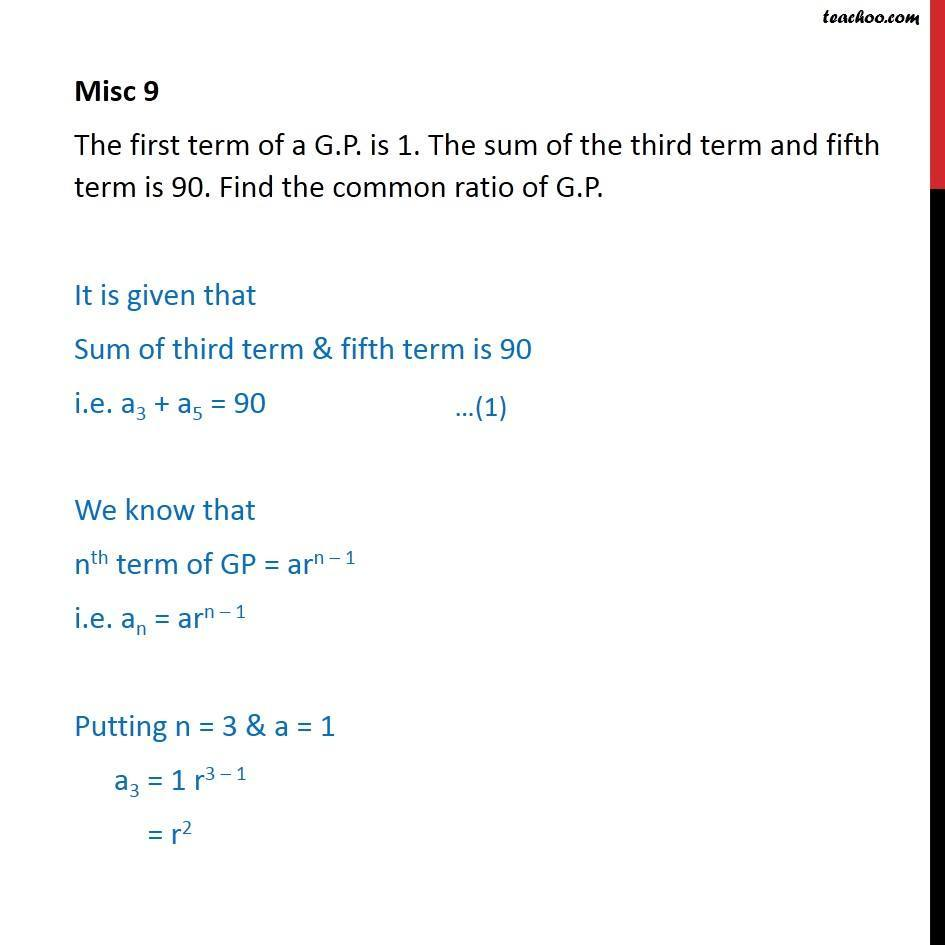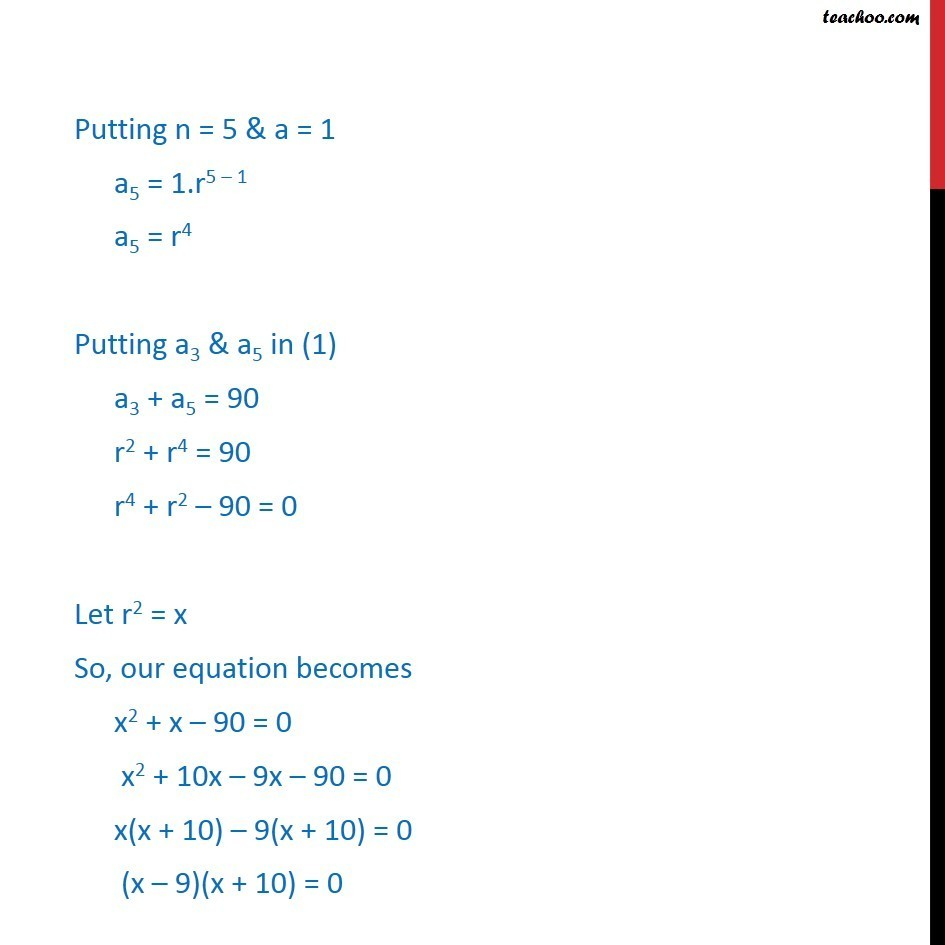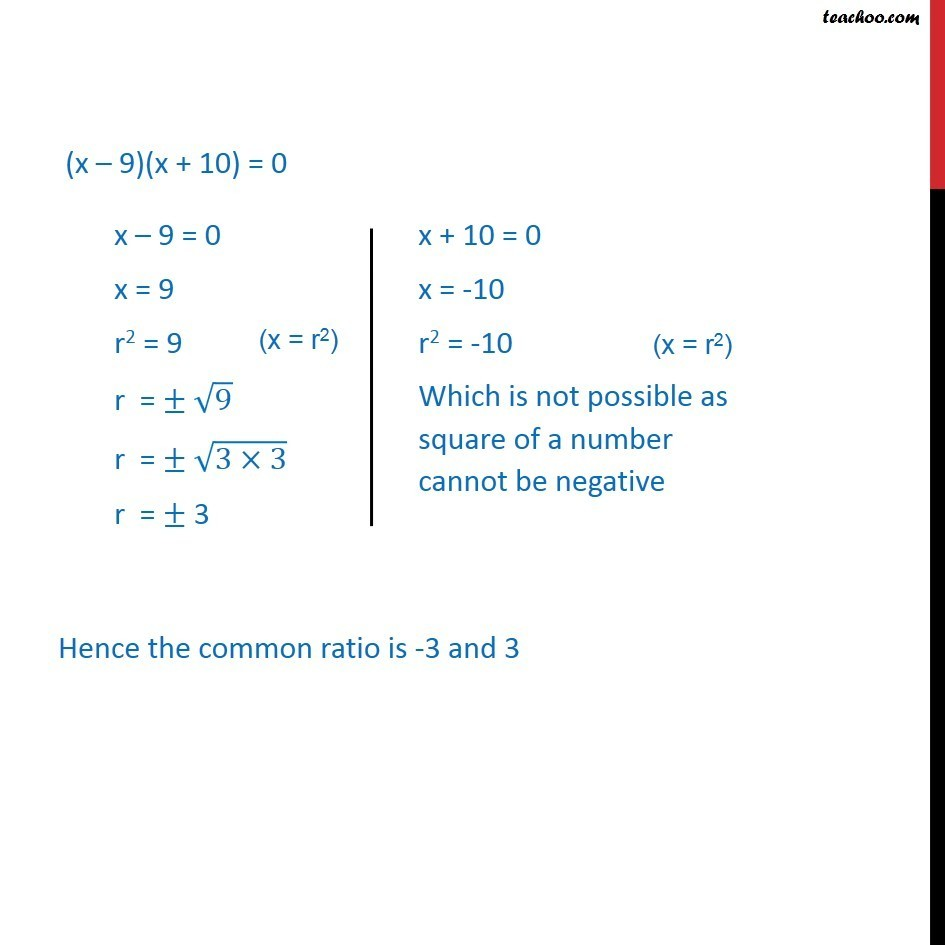Geometric Progression(GP): Formulae based

Chapter 8 Class 11 Sequences and Series
Concept wiseLearn in your speed, with individual attention - Teachoo Maths 1-on-1 Class

### Transcript

Misc 3 The first term of a G.P. is 1. The sum of the third term and fifth term is 90. Find the common ratio of G.P. It is given that Sum of third term & fifth term is 90 i.e. a3 + a5 = 90 We know that nth term of GP = arn – 1 i.e. an = arn – 1 Putting n = 3 & a = 1 a3 = 1 r3 – 1 = r2 Putting n = 5 & a = 1 a5 = 1.r5 – 1 a5 = r4 Putting a3 & a5 in (1) a3 + a5 = 90 r2 + r4 = 90 r4 + r2 – 90 = 0 Let r2 = x So, our equation becomes x2 + x – 90 = 0 x2 + 10x – 9x – 90 = 0 x(x + 10) – 9(x + 10) = 0 (x – 9)(x + 10) = 0 (x – 9)(x + 10) = 0 Hence the common ratio is -3 and 3